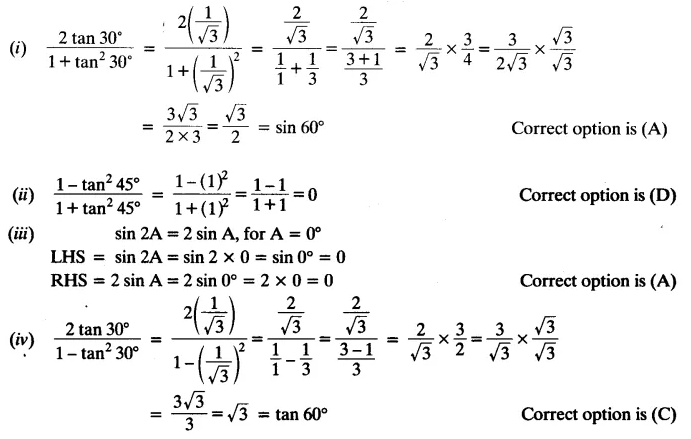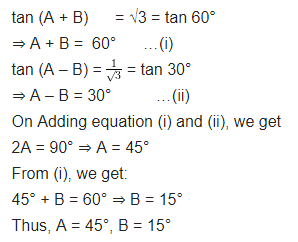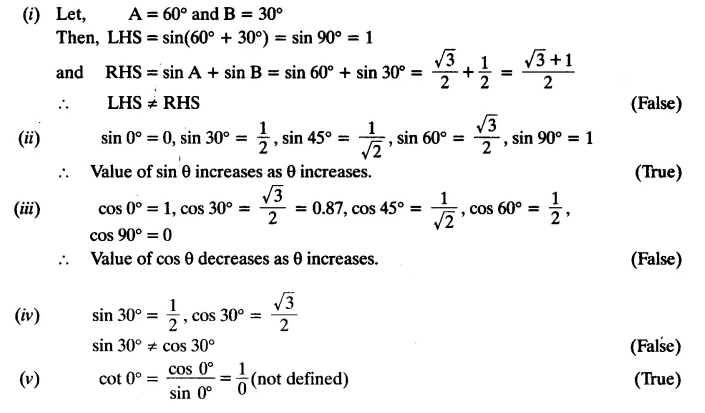# NCERT Solutions For Class 10 Maths Chapter 8 Introduction to Trigonometry Ex 8.2

Get Free NCERT Solutions for Class 10 Maths Chapter 8 Ex 8.2 Introduction to Trigonometry. Class 10 Maths NCERT Solutions are extremely helpful while doing homework. Exercise 8.2 Class 10 Maths NCERT Solutions were prepared by Experienced ncert-books.in Teachers. Detailed answers of all the questions in Chapter 8 Maths Class 10 Coordinate Geometry Exercise 8.2 Provided in NCERT Textbook.NCERT Solutions For Class 10 Maths Chapter 8 Introduction to Trigonometry Ex 8.2

Question 1.
Evaluate the following:Solution:Question 2.
Choose the correct option and justify your choice:Solution:Question 3.
If tan (A + B) = √3 and tan (A – B) =$\frac { 1 }{ \surd 3 }$; 0° < A + B ≤ 90°; A > B, find A and B.
Solution:Question 4.
State whether the following statements are true or false. Justify your answer.
(i) sin (A + B) = sin A + sin B.
(ii) The value of sin θ increases as θ increases.
(iii) The value of cos θ increases as θ increases.
(iv) sin θ = cos θ for all values of θ.
(v) cot A is not defined for A = 0°.
Solution:+